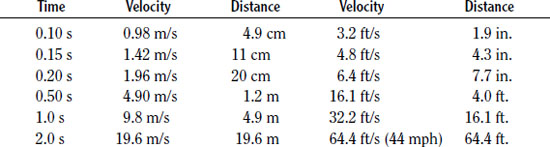# How do the speed and position of a dropped object vary with time?

As long as the only force is the gravitational force, then the acceleration is the acceleration due to gravity, g. At Earth’s surface the value is 9.8 m/s2. (In the English system g = 32.2 ft/s2.) If the object is dropped from rest at time t = 0, then the velocity at a future time t is simply v = gt. That is, the velocity increases by 9.8 m/s each second. If we measure the distance fallen from the position where it was dropped, then at time t it has fallen a distance d = 1/2 gt2. The following table shows velocity and distance fallen for some selected times.Close

This is a web preview of the "The Handy Physics Answer Book" app. Many features only work on your mobile device. If you like what you see, we hope you will consider buying. Get the App